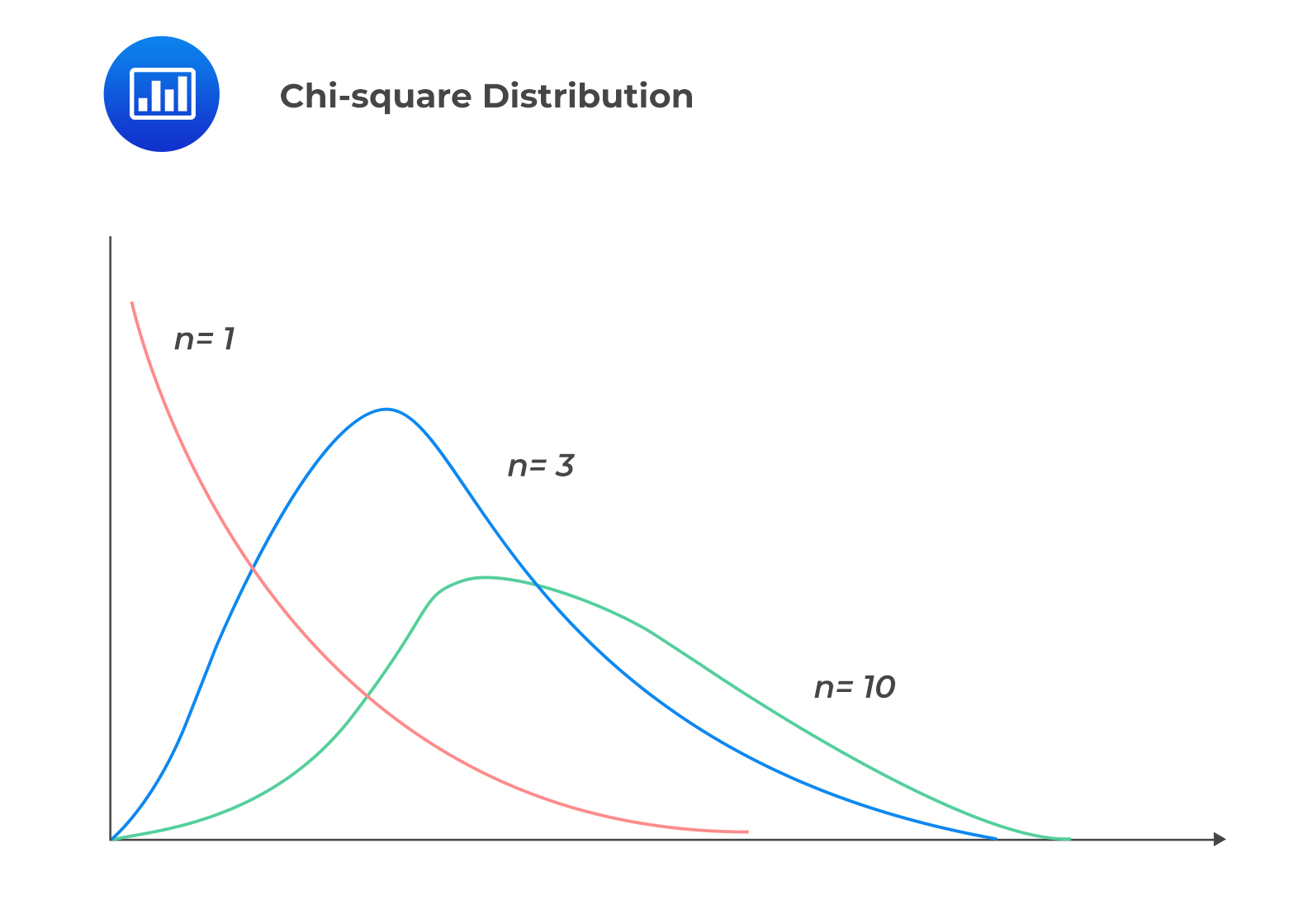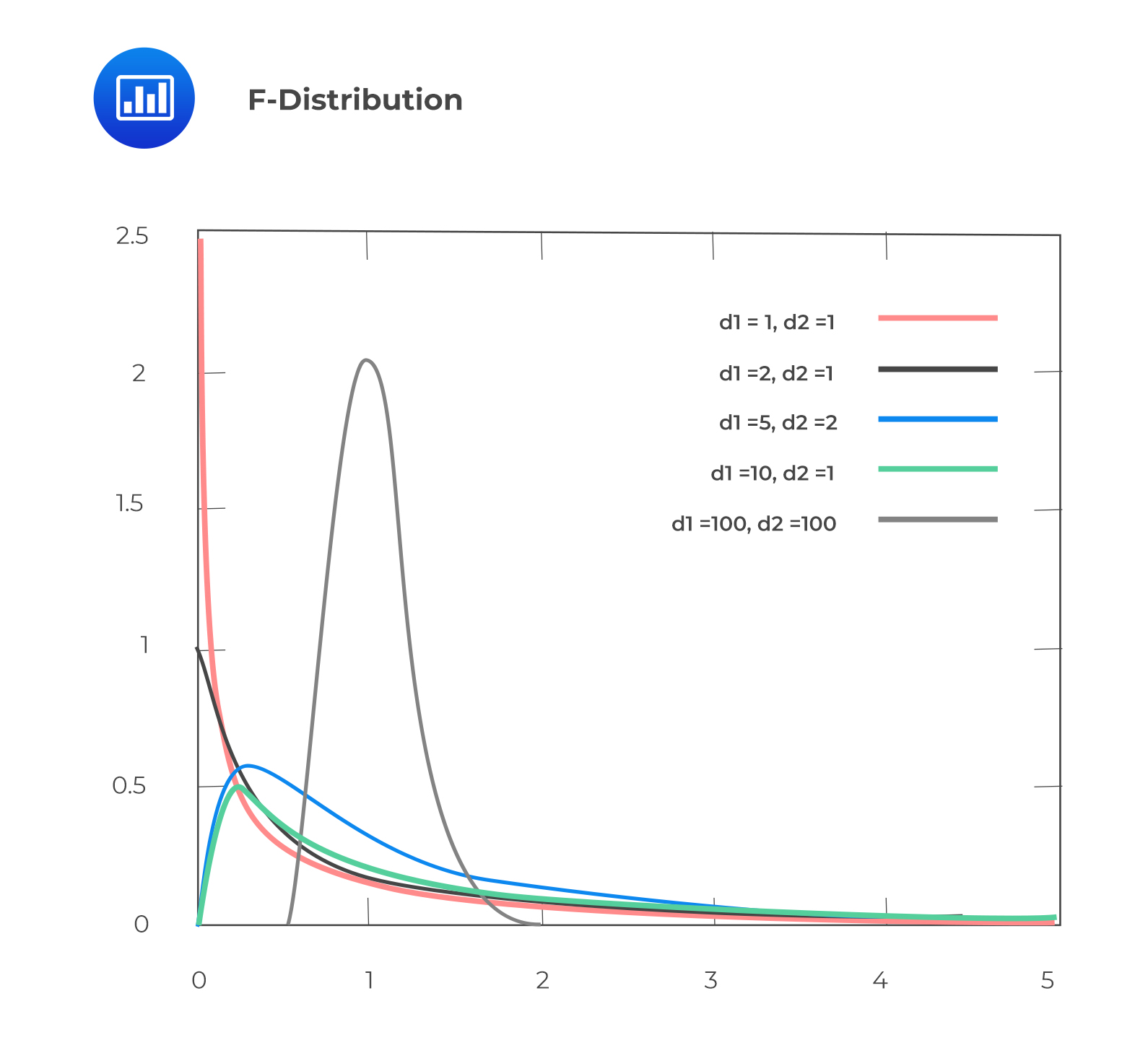# Chi-square and F-Distributions

## Chi-square Distribution

A chi-square distribution is an asymmetrical family of distributions. A chi-square distribution with $$v$$ degrees of freedom is the distribution of the sum of the squares of  $$v$$ independent standard normally distributed random variables. Intuitively, chi-square distributions take only non-negative random variables.

A chi-square distribution is used to test the variance of a population that is distributed normally.

In a summary, the following are the properties of a chi-square distribution:

• A chi-square distribution is a non-symmetrical distribution (skewed to the right).
• A chi-square distribution is defined by one parameter: Degrees of freedom (df), $$v = n – 1$$.
• A chi-square distribution is the sum of the squares of $$k$$ independent standard normally distributed random variables. Hence, it is a non-negative distribution.
• For each degree of freedom, there are different chi-square distributions.
• The shape of a chi-square distribution changes with the change in the degrees of freedom. The more the degrees of free increase, the more the distribution assumes the shape of a standard normal distribution.## F-Distribution

An F-distribution is used to test the equality of variances of two normally distributed populations from two independent random samples.

The following are the properties of an F-distribution:

• An F-distribution is an asymmetrical distribution (skewed to the right).
• An F-distribution is defined by two parameters, i.e., degrees of freedom of the numerator ( $$m$$) and degrees of freedom of the denominator ( $$n$$).
• Like a chi-square distribution, an F-distribution can only have positive values.
• As the degrees of freedom for the numerator and the denominator increase, the F-distribution approximates the normal distribution.### Relationship between the Chi-square and F-distributions

The F-distribution is the ratio of two chi-square distributions with degrees of freedom $$m$$ and  $$n$$, respectively, where each chi-square has first been divided by its degrees of freedom, i.e.,

$$F=\frac{\left(\frac{\chi_{1}^{2}}{m}\right)}{\left(\frac{\chi_{2}^{2}}{n}\right)}$$

Where  $$m$$ is the numerator degrees of freedom and  $$n$$ is the denominator degrees of freedom.

## Question

Which of the following are most likely common characteristics of F-distribution and chi-square distribution?

1. Both can take only positive value.
2. Both are defined by two parameters.
3. Both are negatively skewed distributions.

Solution

Both F-distribution and chi-square distribution can only take non-negative values.

B is incorrect. A chi-square distribution is defined by one parameter (i.e., n-1 degrees of freedom), while an F-distribution is defined by parameters, i.e., degrees of freedom of the numerator (m) and degrees of freedom of the denominator (n).

C is incorrect. Both the F-distribution and the chi-square distribution are positively skewed distributions.

Shop CFA® Exam Prep

Offered by AnalystPrepLevel I
Level II
Level III
All Three Levels
Featured Shop FRM® Exam PrepFRM Part I
FRM Part II
FRM Part I & Part II
Learn with Us

Subscribe to our newsletter and keep up with the latest and greatest tips for success
Shop Actuarial Exams PrepExam P (Probability)
Exam FM (Financial Mathematics)
Exams P & FM
Shop GMAT® Exam PrepComplete CourseSergio Torrico
2021-07-23
Excelente para el FRM 2 Escribo esta revisión en español para los hispanohablantes, soy de Bolivia, y utilicé AnalystPrep para dudas y consultas sobre mi preparación para el FRM nivel 2 (lo tomé una sola vez y aprobé muy bien), siempre tuve un soporte claro, directo y rápido, el material sale rápido cuando hay cambios en el temario de GARP, y los ejercicios y exámenes son muy útiles para practicar.diana
2021-07-17
So helpful. I have been using the videos to prepare for the CFA Level II exam. The videos signpost the reading contents, explain the concepts and provide additional context for specific concepts. The fun light-hearted analogies are also a welcome break to some very dry content. I usually watch the videos before going into more in-depth reading and they are a good way to avoid being overwhelmed by the sheer volume of content when you look at the readings.Kriti Dhawan
2021-07-16
A great curriculum provider. James sir explains the concept so well that rather than memorising it, you tend to intuitively understand and absorb them. Thank you ! Grateful I saw this at the right time for my CFA prep.nikhil kumar
2021-06-28
Very well explained and gives a great insight about topics in a very short time. Glad to have found Professor Forjan's lectures.Marwan
2021-06-22
Great support throughout the course by the team, did not feel neglectedBenjamin anonymous
2021-05-10
I loved using AnalystPrep for FRM. QBank is huge, videos are great. Would recommend to a friendDaniel Glyn
2021-03-24
I have finished my FRM1 thanks to AnalystPrep. And now using AnalystPrep for my FRM2 preparation. Professor Forjan is brilliant. He gives such good explanations and analogies. And more than anything makes learning fun. A big thank you to Analystprep and Professor Forjan. 5 stars all the way!michael walshe
2021-03-18
Professor James' videos are excellent for understanding the underlying theories behind financial engineering / financial analysis. The AnalystPrep videos were better than any of the others that I searched through on YouTube for providing a clear explanation of some concepts, such as Portfolio theory, CAPM, and Arbitrage Pricing theory. Watching these cleared up many of the unclarities I had in my head. Highly recommended.